﻿ 有限域上一元多项式环中的Mertens第二定理的二重推广

# 有限域上一元多项式环中的Mertens第二定理的二重推广Double Mertens’ Second Theorem in the Polynomial Ring over a Finite Field

2014年，罗马尼亚数论学家Popa通过引入素数双曲线法得到Mertens第二定理的二重推广： ∑pq≤x1/pq=(ln(lnx)+B)2-ln22+∫0+01/2ln(1-x)/x dx+(ln(lnx)/lnx),其中p，q是素数，B是Mertens常数。在本文中，我们类比Popa的方法，运用Dirichlet双曲线法在有限域上的一元多项式环中得到了Mertens第二定理的二重推广，同时类比Rosen关于代数数域中Mertens第二定理的证明方法，重新证明了有限域上的一元多项式环中的Mertens第二定理。

Abstract: In 2014, by using the prime hyperbola method Popa developed the following double Mertens estimation: ∑pq≤x1/pq=(ln(lnx)+B)2-ln22+∫0+01/2ln(1-x)/x dx+(ln(lnx)/lnx),where p, q are prime numbers and B is Mertens constant. In this paper, by modifying the methods of Popa and based on Dirichlet’s hyperbola method, we generalize the Mertens’ second theorem to the double case on the polynomial ring over a finite field. During this approach, by modifying Rosen’s method on the proof of the Mertens’ second theorem in algebraic number field, we give a new proof of the Mertens’ second theorem on the polynomial ring over a finite field.

1. 引言

L. Euler在1735年发表的论文中证明了下面著名的等式：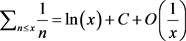, (1)

1874年，德国数学家Mertens进一步得到下面著名的Mertens定理，也叫Mertens第二定理：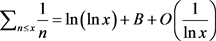, (2)

2014年，罗马尼亚数论学家Popa  通过引入素数双曲线法，即利用如下公式：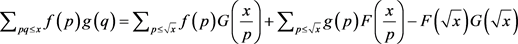, (3)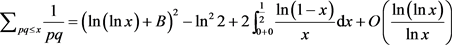, (4)

Mertens第二定理除了整数环中的上述推广和改进之外，人们在有限域上的一元多项式环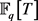中也类比得到了较好的结果。

1979年，南非数论学家Knopfmacher在他编写的书  中，通过引入多项式环上的范数，给出了Mertens第二定理在中的类比：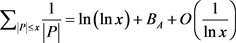, (5)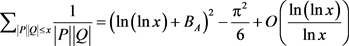, (6)

2. 预备知识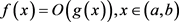.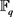是q元有限域，记有限域上的一元多项式环。.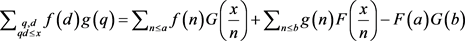,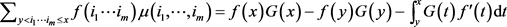,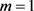时，上述引理就是Abel求和公式。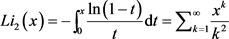,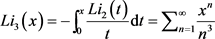.

(i) 对任意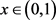，下面等式成立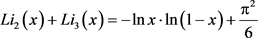.

(ii)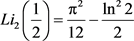.

(iii)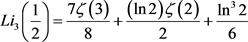.

3. 定理1的证明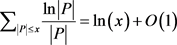.,.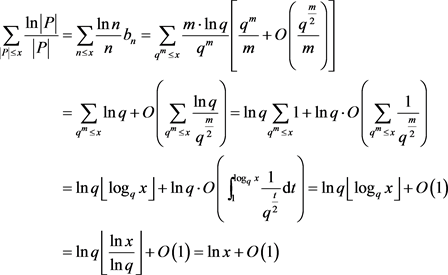,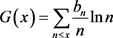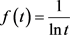.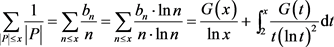. (7), (8).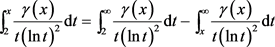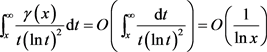,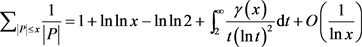.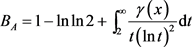,

4. 定理2的证明

(i)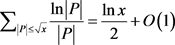.

(ii) 当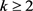时，我们得到(9)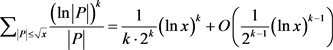. (10)

(ii) 令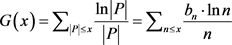,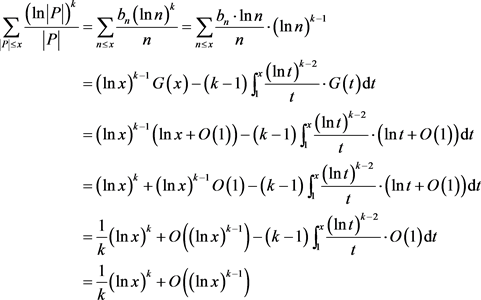.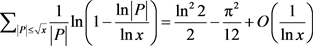., (11)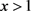时，我们得到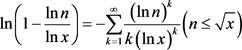., (12). (13)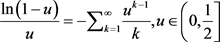,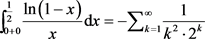.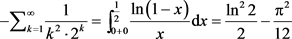. (14)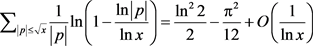.,,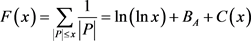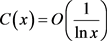..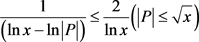,... (15)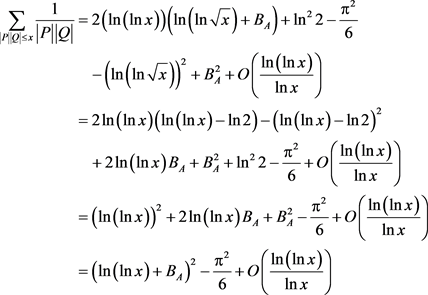.

 Rosen, M. (1999) A Generation of Mertens’ Theorem. Journal of the Ramanujan Mathematical Society, 14, 1-19.

 Apostol, T.M. (2013) Introduction to Analytic Number Theory. Springer Science & Business Media, New York.

 Everest, G. and Ward, T. (2006) An Introduction to Number Theory. Springer Science & Business Media, New York.

 潘承彪, 潘承洞. 素数定理的初等证明[M]. 第二版. 哈尔滨: 哈尔滨工业大学出版社, 2017.

 Popa, D. (2014) A Double Mertens Type Evaluation. Journal of Mathematical Analysis and Applications, 409, 1159-1163.
https://doi.org/10.1016/j.jmaa.2013.07.058

 Knopfmacher, J. (1979) Analytic Arithmetic of Algebraic Function Fields. Marcel Dekker Incorporated, New York.

 潘承洞, 于秀源. 阶的估计基础[M]. 北京: 高等教育出版社, 2015.

 Rosen, M. (2013) Number Theory in Function Fields. Springer Science & Business Media, New York.

 Bănescu, M. and Popa, D. (2018) A Multiple Abel Summation Formula and Asymptotic Evaluations for Multiple Sums. International Journal of Number Theory, 14, 1197-1210.
https://doi.org/10.1142/S1793042118500732

 Lewin, L. (1981) Polylogarithms and Associated Functions. North Holland, New York.

Top